Courses

# Pressure, Elevation and Total Heads Civil Engineering (CE) Notes | EduRev

## Civil Engineering (CE) : Pressure, Elevation and Total Heads Civil Engineering (CE) Notes | EduRev

The document Pressure, Elevation and Total Heads Civil Engineering (CE) Notes | EduRev is a part of the Civil Engineering (CE) Course Soil Mechanics.
All you need of Civil Engineering (CE) at this link: Civil Engineering (CE)

In soils, the interconnected pores provide passage for water. A large number of such flow paths act together, and the average rate of flow is termed the coefficient of permeability, or just permeability. It is a measure of the ease that the soil provides to the flow of water through its pores.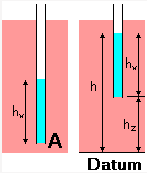At point A, the pore water pressure (u) can be measured from the height of water in a standpipe located at that point.

The height of the water column is the pressure head (hw).
hw = u/gw

To identify any difference in pore water pressure at different points, it is necessary to eliminate the effect of the points of measurement. With this in view, a datum is required from which locations are measured.

The elevation head (hz) of any point is its height above the datum line. The height of water level in the standpipe above the datum is the piezometric head (h).

h = hzhw

Total head consists of three components: elevation head, pressure head, and velocity head. As seepage velocity in soils is normally low, velocity head is ignored, and total head becomes equal to the piezometric head. Due to the low seepage velocity and small size of pores, the flow of water in the pores is steady and laminar in most cases. Water flow takes place between two points in soil due to the difference in total heads.

Darcy's Law

Darcy's law states that there is a linear relationship between flow velocity (v) and hydraulic gradient (i) for any given saturated soil under steady laminar flow conditions.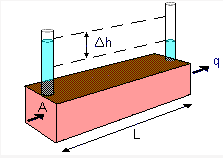If the rate of flow is q (volume/time) through cross-sectional area (A) of the soil mass, Darcy's Law can be expressed as

v = q/A = k.i

where permeability of the soil
i = Dh/L
Dh = difference in total heads
Llength of the soil mass

The flow velocity (v) is also called the Darcian velocity or the superficial velocity. It is different from the actual velocity inside the soil pores, which is known as the seepage velocity, vS. At the particulate level, the water follows a tortuous path through the pores. Seepage velocity is always greater than the superficial velocity, and it is expressed as: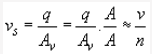where AV = Area of voids on a cross section normal to the direction of flow
n = porosity of the soil

Permeability of Different Soils

Permeability (k) is an engineering property of soils and is a function of the soil type. Its value depends on the average size of the pores and is related to the distribution of particle sizes, particle shape and soil structure. The ratio of permeabilities of typical sands/gravels to those of typical clays is of the order of 106. A small proportion of fine material in a coarse-grained soil can lead to a significant reduction in permeability.

For different soil types as per grain size, the orders of magnitude for permeability are as follows: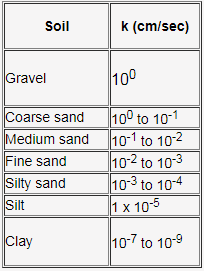Factors affecting Permeability

In soils, the permeant or pore fluid is mostly water whose variation in property is generally very less. Permeability of all soils is strongly influenced by the density of packing of the soil particles, which can be represented by void ratio (e) or porosity (n).

For Sands
In sands, permeability can be empirically related to the square of some representative grain size from its grain-size distribution. For filter sands, Allen Hazen in 1911 found that k » 100 (D10)2 cm/s where D10= effective grain size in cm.

Different relationships have been attempted relating void ratio and permeability, such as k µ e3/(1+e), and k µ e2. They have been obtained from the Kozeny-Carman equation for laminar flow in saturated soils.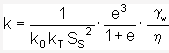where ko and kT are factors depending on the shape and tortuosity of the pores respectively, SS is the surface area of the solid particles per unit volume of solid material, and gw and h are unit weight and viscosity of the pore water. The equation can be reduced to a simpler form as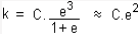For Silts and Clays
For silts and clays, the Kozeny-Carman equation does not work well, and log k versus e plot has been found to indicate a linear relationship.

For clays, it is typically found that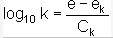where Ckis the permeability change index and eis a reference void ratio.

Offer running on EduRev: Apply code STAYHOME200 to get INR 200 off on our premium plan EduRev Infinity!

## Soil Mechanics

31 videos|48 docs|42 tests

,

,

,

,

,

,

,

,

,

,

,

,

,

,

,

,

,

,

,

,

,

,

,

,

;